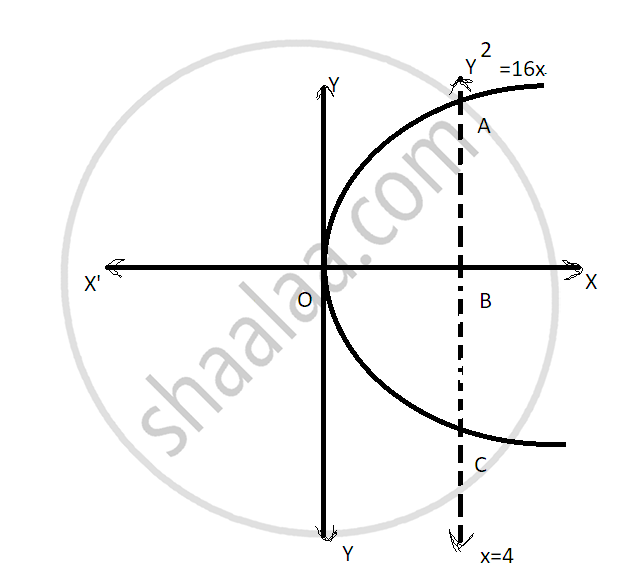# Find the Area of the Region Bounded by the Parabola Y2 = 16x and the Line X = 4. - Mathematics and Statistics

Sum

Find the area of the region bounded by the parabola y2 = 16x and the line x = 4.

#### Solution

The region bounded by the parabola y^2 = 16x and
the line x = 4 is the area OACO
The area OACO is symmetrical about x-axis
Area of OACO = 2(Area of OAB)Area of OACO = 2[int_0^4y dx]

=2int_0^4 4sqrtx   dx

=8((x^(3/2 )/(3/2))_0^4

=16/3((4)3/2)

=16/3(8)=128/3

Therefore, the required area is 128/3sq. units.

#### Notes

Concept: Inverse of Matrix
Is there an error in this question or solution?